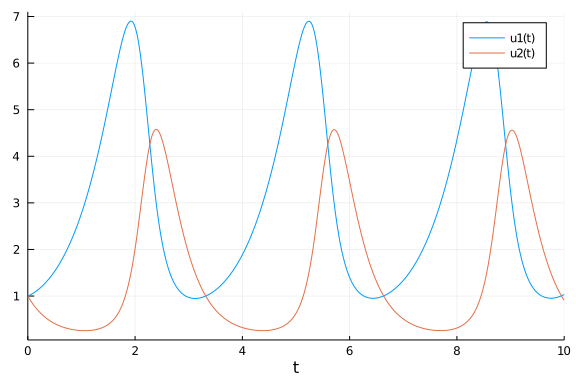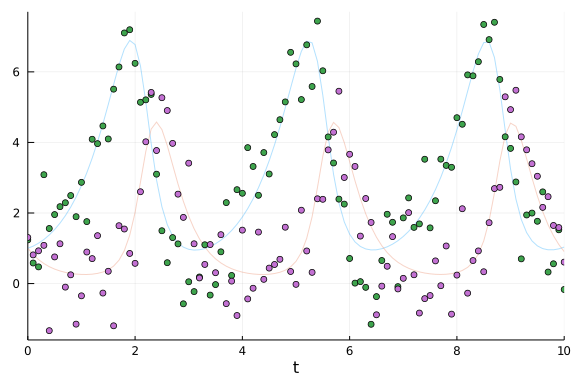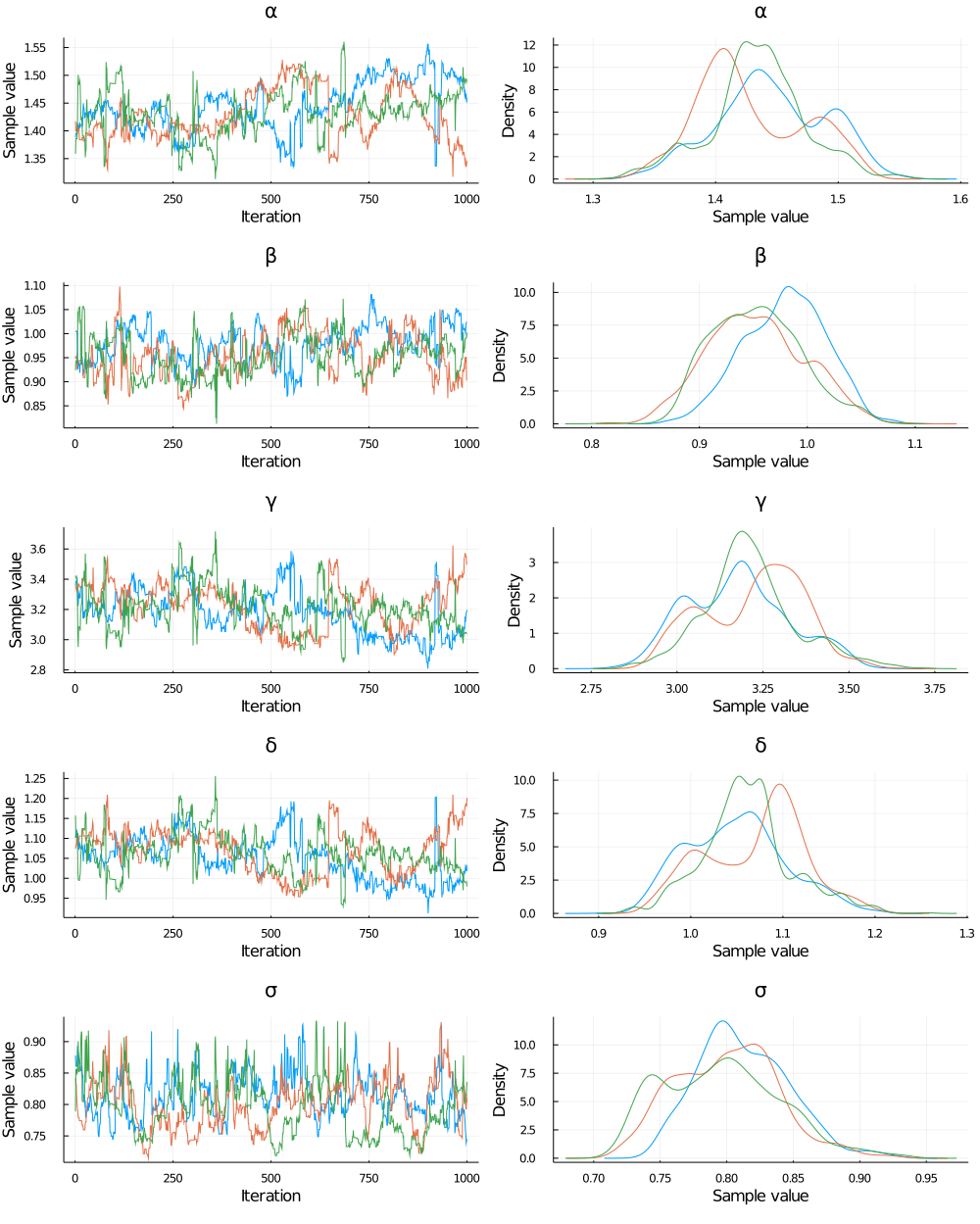# GPU-Accelerated Data-Driven Bayesian Uncertainty Quantification with Koopman Operators

##### Chris Rackauckas

What if you have data and a general model and would like to evaluate the probability that the fitted model outcomes would have had a given behavior? The purpose of this tutorial is to demonstrate a fast workflow for doing exactly this. It composes together a few different pieces of the SciML ecosystem:

1. Parameter estimation with uncertainty with Bayesian differential equations by integrating the differentiable differential equation solvers with the Turing.jl library.

2. Fast calculation of probabilistic estimates of differential equation solutions with parametric uncertainty using the Koopman expectation.

3. GPU-acceleration of batched differential equation solves.

Let's dive right in.

## Bayesian Parameter Estimation with Uncertainty

Let's start by importing all of the necessary libraries:

using Turing, Distributions, DifferentialEquations
using MCMCChains, Plots, StatsPlots
using Random
using DiffEqUncertainty
using KernelDensity, DiffEqUncertainty
using Cuba, DiffEqGPU

Random.seed!(1);


For this tutorial we will use the Lotka-Volterra equation:

function lotka_volterra(du,u,p,t)
@inbounds begin
x = u
y = u
α = p
β = p
γ = p
δ = p
du = (α - β*y)*x
du = (δ*x - γ)*y
end
end
p = [1.5, 1.0, 3.0, 1.0]
u0 = [1.0,1.0]
prob1 = ODEProblem(lotka_volterra,u0,(0.0,10.0),p)
sol = solve(prob1,Tsit5())
plot(sol)From the Lotka-Volterra equation we will generate a dataset with known parameters:

sol1 = solve(prob1,Tsit5(),saveat=0.1)

retcode: Success
Interpolation: 1st order linear
t: 101-element Array{Float64,1}:
0.0
0.1
0.2
0.3
0.4
0.5
0.6
0.7
0.8
0.9
⋮
9.2
9.3
9.4
9.5
9.6
9.7
9.8
9.9
10.0
u: 101-element Array{Array{Float64,1},1}:
[1.0, 1.0]
[1.0610780673356455, 0.8210842775886171]
[1.1440276717257598, 0.6790526689784505]
[1.2491712125724483, 0.5668931465841183]
[1.377644570563638, 0.4788129513795158]
[1.5312308177480447, 0.4101564670858799]
[1.7122697558185596, 0.3572654487973182]
[1.923578275830245, 0.31734720616244183]
[2.16839108970141, 0.28838884378747953]
[2.45025066713923, 0.26905370939591183]
⋮
[1.8172822873986514, 4.064946536272397]
[1.4427612797125045, 3.5397375166871554]
[1.2089080986913683, 2.991454947925124]
[1.0685925962278562, 2.482072872915716]
[0.9910229662215924, 2.0372445308562717]
[0.9574213545848079, 1.6632055422875025]
[0.9569793998644455, 1.3555870044265412]
[0.9835609175876892, 1.106286801228109]
[1.0337581393337212, 0.9063703701433541]


Now let's assume our dataset should have noise. We can add this noise in and plot the noisy data against the generating set:

odedata = Array(sol1) + 0.8 * randn(size(Array(sol1)))
plot(sol1, alpha = 0.3, legend = false); scatter!(sol1.t, odedata')Now let's assume that all we know is the data odedata and the model form. What we want to do is use the data to inform us of the parameters, but also get a probabilistic sense of the uncertainty around our parameter estimate. This is done via Bayesian estimation. For a full look at Bayesian estimation of differential equations, look at the Bayesian differential equation tutorial from Turing.jl.

Following that tutorial, we choose a set of priors and perform NUTS sampling to arrive at the MCMC chain:

Turing.setadbackend(:forwarddiff)

@model function fitlv(data, prob1)
σ ~ InverseGamma(2, 3) # ~ is the tilde character
α ~ truncated(Normal(1.5,0.5),1.0,2.0)
β ~ truncated(Normal(1.2,0.5),0.5,1.5)
γ ~ truncated(Normal(3.0,0.5),2,4)
δ ~ truncated(Normal(1.0,0.5),0.5,1.5)

p = [α,β,γ,δ]
prob = remake(prob1, p=p)
predicted = solve(prob,Tsit5(),saveat=0.1)

for i = 1:length(predicted)
data[:,i] ~ MvNormal(predicted[i], σ)
end
end

model = fitlv(odedata, prob1)

# This next command runs 3 independent chains without using multithreading.
chain = mapreduce(c -> sample(model, NUTS(.45),1000), chainscat, 1:3)

Chains MCMC chain (1000×17×3 Array{Float64,3}):

Iterations        = 1:1000
Thinning interval = 1
Chains            = 1, 2, 3
Samples per chain = 1000
parameters        = α, β, γ, δ, σ
internals         = acceptance_rate, hamiltonian_energy, hamiltonian_energy
_error, is_accept, log_density, lp, max_hamiltonian_energy_error, n_steps,
nom_step_size, numerical_error, step_size, tree_depth

Summary Statistics
parameters      mean       std   naive_se      mcse       ess      rhat
Symbol   Float64   Float64    Float64   Float64   Float64   Float64

α    1.4360    0.0433     0.0008    0.0060   25.6755    1.1135
β    0.9617    0.0430     0.0008    0.0053   34.3566    1.1060
γ    3.2048    0.1439     0.0026    0.0192   29.3296    1.0979
δ    1.0607    0.0528     0.0010    0.0072   29.1891    1.0991
σ    0.8030    0.0398     0.0007    0.0045   53.8220    1.0849

Quantiles
parameters      2.5%     25.0%     50.0%     75.0%     97.5%
Symbol   Float64   Float64   Float64   Float64   Float64

α    1.3528    1.4074    1.4333    1.4652    1.5175
β    0.8827    0.9306    0.9618    0.9929    1.0441
γ    2.9452    3.0988    3.2037    3.3000    3.4929
δ    0.9664    1.0221    1.0620    1.0951    1.1673
σ    0.7320    0.7741    0.8015    0.8292    0.8872


This chain gives a discrete approximation to the probability distribution of our desired quantites. We can plot the chains to see this distributions in action:

plot(chain)Great! From our data we have arrived at a probability distribution for the our parameter values.

## Evaluating Model Hypotheses with the Koopman Expectation

Now let's try and ask a question: what is the expected value of x (the first term in the differential equation) at time t=10 given the known uncertainties in our parameters? This is a good tutorial question because all other probabilistic statements can be phrased similarly. Asking a question like, "what is the probability that x(T) > 1 at the final time T?", can similarly be phrased as an expected value (probability statements are expected values of characteristic functions which are 1 if true 0 if false). So in general, the kinds of questions we want to ask and answer are expectations about the solutions of the differential equation.

The trivial to solve this problem is to sample 100,000 sets of parameters from our parameter distribution given by the Bayesian estimation, solve the ODE 100,000 times, and then take the average. But is 100,000 ODE solves enough? Well it's hard to tell, and even then, the convergence of this approach is slow. This is the Monte Carlo approach and it converges to the correct answer by sqrt(N). Slow.

However, the Koopman expectation can converge with much fewer points, allowing the use of higher order quadrature methods to converge exponentially faster in many cases. To use the Koopman expectation functionality provided by DiffEqUncertainty.jl, we first need to define our observable function g. This function designates the thing about the solution we wish to calculate the expectation of. Thus for our question "what is the expected value of xat time t=10?", we would simply use:

function g(sol)
sol[1,end]
end

g (generic function with 1 method)


Now we need to use the expectation call, where we need to provide our initial condition and parameters as probability distirbutions. For this case, we will use the same constant u0 as before. But, let's turn our Bayesian MCMC chains into distributions through kernel density estimation (the plots of the distribution above are just KDE plots!).

p_kde = [kde(vec(Array(chain[:α]))),kde(vec(Array(chain[:β]))),
kde(vec(Array(chain[:γ]))),kde(vec(Array(chain[:δ])))]

4-element Array{KernelDensity.UnivariateKDE{StepRangeLen{Float64,Base.Twice
Precision{Float64},Base.TwicePrecision{Float64}}},1}:
KernelDensity.UnivariateKDE{StepRangeLen{Float64,Base.TwicePrecision{Float
64},Base.TwicePrecision{Float64}}}(1.2819238573935134:0.0001513746244249977
:1.5917877135914837, [1.2503827098164777e-5, 1.255480742923254e-5, 1.267922
2669120804e-5, 1.2877536693878255e-5, 1.3150535124850649e-5, 1.349932675420
7175e-5, 1.3925345814236323e-5, 1.4430355088634883e-5, 1.5016449860461023e-
5, 1.568606270474504e-5  …  1.6162385334084206e-5, 1.54330429666949e-5, 1.4
790495180716512e-5, 1.4231876743098226e-5, 1.3754677651744984e-5, 1.3356735
79028196e-5, 1.303623049053737e-5, 1.2791676995638213e-5, 1.262192181666899
e-5, 1.2526138974122691e-5])
KernelDensity.UnivariateKDE{StepRangeLen{Float64,Base.TwicePrecision{Float
64},Base.TwicePrecision{Float64}}}(0.7813328038630115:0.0001699027135499333
5:1.129123658499725, [1.0952195927949049e-5, 1.1030910327392007e-5, 1.11882
41592741122e-5, 1.1425008563215044e-5, 1.1742439271600347e-5, 1.21421744863
07056e-5, 1.2626272384075321e-5, 1.3197214371984956e-5, 1.3857912064096922e
-5, 1.4611715374535095e-5  …  1.460012404246036e-5, 1.3847895343366279e-5,
1.3188649366821514e-5, 1.2619053473855724e-5, 1.2136212244287514e-5, 1.1737
659540544954e-5, 1.1421351695517501e-5, 1.1185661827423088e-5, 1.1029375312
832101e-5, 1.0951686391064142e-5])
KernelDensity.UnivariateKDE{StepRangeLen{Float64,Base.TwicePrecision{Float
64},Base.TwicePrecision{Float64}}}(2.704075727382457:0.0005456915762053855:
3.821106383874881, [3.2868758819137867e-6, 3.309017689190341e-6, 3.35295899
6671873e-6, 3.4189140643015037e-6, 3.5072022273041625e-6, 3.618248789694078
5e-6, 3.752586195782115e-6, 3.910855473021613e-6, 4.093807958627238e-6, 4.3
02307299974828e-6  …  4.292022644875049e-6, 4.084920701238892e-6, 3.9032563
54090279e-6, 3.7461813835182323e-6, 3.612958888538076e-6, 3.502961436654961
e-6, 3.415669480727468e-6, 3.3506700490015806e-6, 3.307655704526402e-6, 3.2
864237783369887e-6])
KernelDensity.UnivariateKDE{StepRangeLen{Float64,Base.TwicePrecision{Float
64},Base.TwicePrecision{Float64}}}(0.8735922924134194:0.0002055200760229648
:1.2942918880324283, [8.930132326945284e-6, 8.992083831316222e-6, 9.1162496
84026911e-6, 9.303254405956807e-6, 9.554036559222823e-6, 9.869851314903144e
-6, 1.025227389650496e-5, 1.0703203848105858e-5, 1.1224870170245538e-5, 1.1
819837285376167e-5  …  1.1818668460172876e-5, 1.12238676836518e-5, 1.070235
251582341e-5, 1.025156076783773e-5, 9.869265502615576e-6, 9.553569082609847
e-6, 9.302898083818345e-6, 9.115999025754995e-6, 8.991934969615478e-6, 8.93
0082961045027e-6])


Now that we have our observable and our uncertainty distributions, let's calculate the expected value:

expect = expectation(g, prob1, u0, p_kde, Koopman(), Tsit5(), quadalg = CubaCuhre())

u: 1.1120850335713364


Note how that gives the expectation and a residual for the error bound!

expect.resid

1-element Array{Float64,1}:
0.011060823024648208


### GPU-Accelerated Expectations

Are we done? No, we need to add some GPUs! As mentioned earlier, probability calculations can take quite a bit of ODE solves, so let's parallelize across the parameters. DiffEqGPU.jl allows you to GPU-parallelize across parameters by using the Ensemble interface. Note that you do not have to do any of the heavy lifting: all of the conversion to GPU kernels is done automaticaly by simply specifying EnsembleGPUArray as the ensembling method. For example:

function lotka_volterra(du,u,p,t)
@inbounds begin
x = u
y = u
α = p
β = p
γ = p
δ = p
du = (α - β*y)*x
du = (δ*x - γ)*y
end
end
p = [1.5, 1.0, 3.0, 1.0]
u0 = [1.0,1.0]
prob = ODEProblem(lotka_volterra,u0,(0.0,10.0),p)
prob_func = (prob,i,repeat) -> remake(prob,p=rand(Float64,4).*p)
monteprob = EnsembleProblem(prob, prob_func = prob_func, safetycopy=false)
@time sol = solve(monteprob,Tsit5(),EnsembleGPUArray(),trajectories=10_000,saveat=1.0f0)

36.403861 seconds (75.95 M allocations: 5.218 GiB, 2.51% gc time)
EnsembleSolution Solution of length 10000 with uType:
DiffEqBase.ODESolution{Float64,2,uType,Nothing,Nothing,Array{Float64,1},rat
eType,DiffEqBase.ODEProblem{Array{Float64,1},Tuple{Float64,Float64},true,Ar
ray{Float64,1},DiffEqBase.ODEFunction{true,typeof(Main.##WeaveSandBox#253.l
otka_volterra),LinearAlgebra.UniformScaling{Bool},Nothing,Nothing,Nothing,N
othing,Nothing,Nothing,Nothing,Nothing,Nothing,Nothing,Nothing,Nothing},Bas
e.Iterators.Pairs{Union{},Union{},Tuple{},NamedTuple{(),Tuple{}}},DiffEqBas
e.StandardODEProblem},OrdinaryDiffEq.Tsit5,IType,DiffEqBase.DEStats} where
IType where rateType where uType


Let's now use this in the ensembling method. We need to specify a batch for the number of ODEs solved at the same time, and pass in our enembling method. The following is a GPU-accelerated uncertainty quanitified estimate of the expectation of the solution:

expectation(g, prob1, u0, p_kde, Koopman(), Tsit5(), EnsembleGPUArray(), batch=100, quadalg = CubaCuhre())

u: 1-element Array{Float64,1}:
1.1097482321066328